Courses

# NCERT Solutions(Part- 1)- Cubes and Cube Roots Class 8 Notes | EduRev

## Mathematics (Maths) Class 8

Created by: Full Circle

## Class 8 : NCERT Solutions(Part- 1)- Cubes and Cube Roots Class 8 Notes | EduRev

The document NCERT Solutions(Part- 1)- Cubes and Cube Roots Class 8 Notes | EduRev is a part of the Class 8 Course Mathematics (Maths) Class 8.
All you need of Class 8 at this link: Class 8

Question: Find the one’s digit of the cube of each of the following numbers.

(i) 3331 (ii) 8888 (iii) 149 (iv) 1005
(v) 1024 (vi) 77 (vii) 5022 (viii) 53

Solution:

 Number Number ending in Unit’s digit in the cube (i)(ii)(iii)(iv)(v)(vi)(vii)(viii) 333188881491005102477502253 18954723 12954387

Note:

I. There are only 4 numbers, less than 100 which are perfect cubes. They are: 1, 8, 27 and 64.
II. There are only 5 numbers between 100 and 1000 which are perfect cubes. These are: 125, 216, 343, 512 and 729.
III. The least 4 digit perfect cube is 1000.

Question 1. Express the following numbers as the sum of odd numbers using the above pattern?

(a) 63  (b) 83  (c) 73

Solution: (a) n = 6 and (n – 1) = 5

We have

63 = 31 + 33 + 35 + 37 + 39 + 41 = 216

(b) n = 8 and (n – 1) = 7

We have

83 = 57 + 59 + 61 + 63 + 65 + 67 + 69 + 71 = 512

(c) n = 7 and (n – 1) = 6

we have

73 = 43 + 45 + 47 + 49 + 51 + 53 + 55 = 343

Question 2. Consider the following pattern:

23 – 13 = 1 + 2 * 1 * 3
33 – 23 = 1 + 3 * 2 * 3
43 – 33 = 1 + 4 * 3 * 3

Using the above pattern, find the value of the following:

(i) 73 – 63 (ii) 123 – 113 (iii) 203 – 193 (iv) 513 – 503

Solution:

(i) 73 – 63 = 1 + 7 * 6 * 3

= 1 + 126 = 127

(ii) 123 – 113 = 1 + 12 * 11 * 3

= 1 + 396 = 397

(iii) 20– 193 = 1 + 20 * 11 * 3

= 1 + 1140 = 1141

(iv) 513 – 503 = 1 + 51 * 50 * 3

= 1 + 7650 = 7651

Note: In the prime factorisation of any number, if each factor appears three times, then the number is a perfect cube.

Question: Which of the following are perfect cubes?

1. 400           2. 3375
3. 8000         4. 15625
5. 9000         6. 6859
7. 2025         8. 10648

Solution:

1. We have 400 = 2 * 2 * 2 * 2 * 5 * 5

∵ 2 * 5 * 5 remain after grouping in triples.

∴ 400 is not a perfect cube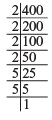2. We have 3375 = 3 * 3 * 3 * 5 * 5 * 5

∵ The prime factors appear in triples.

∴  3375 is a perfect cube.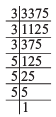3. We have 8000 = 2 * 2 * 2 * 2 * 2 * 2 * 5 * 5 * 5

∵ The prime factors of 8000 can be grouped into triples and no factor is left over.

∴ 8000 is a perfect cube.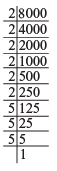4. We have 15625 = 5 * 5 * 5 * 5 * 5 * 5

∵ The prime factors of 15625 can be grouped into triples and no factor is left over.

∴ 15625 is a perfect cube.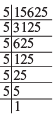5. We have 9000 = 2 * 2 * 2 * 3 * 3 * 5 * 5 * 5

∵ The prime factors of 9000 cannot be grouped into triples (because 3 * 3 are left over).

∴ 9000 is not a perfect cube.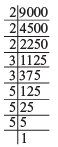6. We have 6859 = 19 * 19 * 19

∵ The prime factors of 6859 can be grouped into triples and no factor is left over.

∴ 6859 is a perfect cube.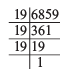7. We have 2025 = 3 * 3 * 3 * 3 * 5 * 5

∵ We do not get triples of prime factors of 2025 and 3 * 5 * 5 are left over.

∴ 2025 is not a perfect cube.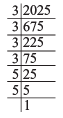8. We have 10648 = 2 * 2 * 2 * 11 * 11 * 11

∵ The prime factors of 10648 can be grouped into triples and no factor is left over.

∴ 10648 is a perfect cube.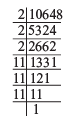Question: Check which of the following are perfect cubes.

(i) 2700 (ii) 16000 (iii) 64000 (iv) 900 (v) 125000
(vi) 36000 (vii) 21600 (viii) 10000 (ix) 27000000 (x) 1000

What pattern do you observe in these perfect cubes?

Solution:

(i) We have 2700 = 2 * 2 * 3 * 3 * 3 * 5 * 5

We do not get complete triples of prime factors, i.e. 2 * 2 and 5 * 5 are left over.

∴ 2700 is not a perfect cube.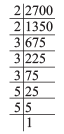(ii) We have 1600 = 2 * 2 * 2 * 2 * 2 * 2 * 5 * 5

After grouping is 3’s we get 5 * 5 which is ungrouped in triples.

∴ 1600 is not a perfect cube.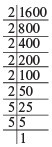(iii) We have 64000 = 2 * 2 * 2 * 2 * 2 * 2 * 2 * 2 * 2 * 5 * 5 * 5

Since, we get groups of triples.

∴ 64000 is a perfect cube.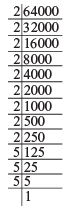(iv) We have 900 = 2 * 2 * 3 * 3 * 5 * 5

which are ungrouped in triples.

∴ 900 is not a perfect cube.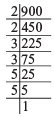(v) We have 125000 = 2 * 2 * 2 * 5 * 5 * 5 * 5 * 5 * 5

As we get all the prime factors in the group of triples.

∴ 125000 is a perfect cube.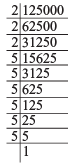(vi) We have 36000 = 2 * 2 * 2 * 2 * 2 * 3 * 3 * 5 * 5 * 5

While grouping the prime factors of 36000 in triples, we are left over with 2 * 2 and 3 * 3.

∴ 36000 is not a perfect cube.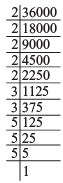(vii) We have 21600 = 2 * 2 * 2 * 2 * 2 * 3 * 3 * 3 * 5 * 5

While grouping the prime factors of 21600 in triples, we are left with 2 * 2 and 5 * 5.

∴ 21600 is not a perfect cube.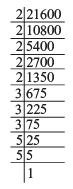(viii) We have 10000 = 2 * 2 * 2 * 2 * 5 * 5 * 5 * 5

While grouping the prime factors into triples, we are left over with 2 and 5.

∴ 10000 is not a perfect cube.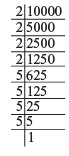(ix) We have 27000000 = 2 * 2 * 2 * 2 * 2 * 2 * 3 * 3 * 3 * 5 * 5 * 5 * 5 * 5 * 5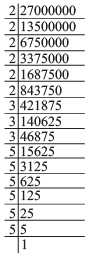Since, all the prime factors of 27000000 appear in groups of triples.

∴ 27000000 is a perfect cube.

(x) We have 1000 = 2 * 2 * 2 * 5 * 5 * 5

Since, all the prime factors of 1000 appear in groups of triples.

∴ 1000 is a perfect cube.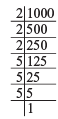Now, in these perfect cubes, we can say that “the number of zeros at the end of a perfect cube must be 3 or a multiple of 3, failing which the number cannot be a perfect cube.”

## Mathematics (Maths) Class 8

217 videos|147 docs|48 tests

,

,

,

,

,

,

,

,

,

,

,

,

,

,

,

,

,

,

,

,

,

;